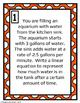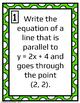# Writing Linear Equations BundleSubject
Resource Type
File Type

Zip

(11 MB|85 pages)
3 Products in this Bundle
3 products
1. These task cards allow students to practice writing equations of parallel and perpendicular lines in slope intercept form. Each task card will ask students to write an equation of a line that is either parallel or perpendicular to a given line and goes through a given point. These cards can be set
2. These task cards allow students to practice writing linear equations when given two points. Each task card will ask students to write an equation of a line that passes through two given points. These cards can be set up around the room allowing students to move through the problems recording their
3. These task cards allow students to practice writing linear equations from word problems. Each task card will ask students to write an equation based on a given situation. Each problem also has an added optional question that asks the student to use their equation to answer a question. These cards
Bonus
1 file
Bundle Description

This bundle includes 3 products to practice writing linear equations. Students will practice writing equations from parallel and perpendicular lines, two points and from real life word problems.

Each Game Contains:

-Teacher instruction page

-Student recording sheet

-Worksheet containing the problems from the 16 task cards

You will also receive a bonus product containing all 48 problems formatted as smaller task cards.

Check out these products for practice with slope:

Calculating Slope Can you catch the mistake

Be sure to follow my store to find out about of new products.

Follow

Don't forget to leave feedback. You will receive TPT credits that can be used on future purchases!

Total Pages
85 pages
Included
Teaching Duration
90 minutes
Report this Resource to TpT
Reported resources will be reviewed by our team. Report this resource to let us know if this resource violates TpT’s content guidelines.